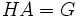# Abelian-completed subgroup

This article defines a subgroup property: a property that can be evaluated to true/false given a group and a subgroup thereof, invariant under subgroup equivalence. View a complete list of subgroup properties[SHOW MORE]

## Definition

### Symbol-free definition

A subgroup of a group is termed Abelian-completed if there is an Abelian subgroup such that their product is the whole group.

### Definition with symbols

A subgroup$H$ of a group$G$ is termed Abelian-completed if there is an Abelian subgroup$A$ such that$HA = G$.

## Metaproperties

### Upward-closedness

This subgroup property is upward-closed: if a subgroup satisfies the property in the whole group, every intermediate subgroup also satisfies the property in the whole group
View other upward-closed subgroup properties

If$H$ and$A$ generate$G$, then so do$K$ and$A$ for any$K$ containing$H$. Hence, the property of being Abelian-completed is upward-closed.